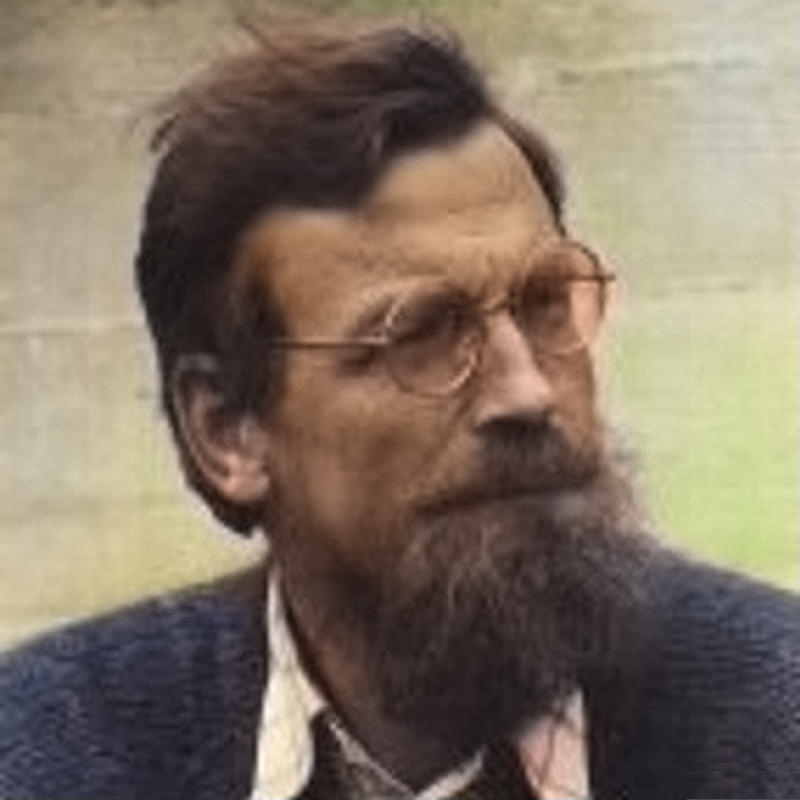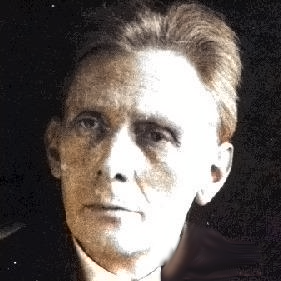# Nonstandard Proof of Furstenberg's Recurrence theorem

Oliver Knill
Here is the document from 1995 as a PDF

 We apply the language of nonstandard analysis (IST) to topological dynamics. As an illustration, we translate the proof of Furstenbergs multiple recurrence theorem into the framework of nonstandard analysis. That proof had been presented in a Specker seminar around 1989-1990, this document was TeXed up in English 1995 while at Caltech.

 As a sophomore math student at ETH, I got a copy of Chinchin's "Drei Perlen der Zahlentheorie": The van der Waerden Theorem is: If the natural numbers is partitioned into finitely many sets, one of them contains an arbitrary long arithmetic sequence. For example, if we look at the set A of integers for which the n'th digit of π is even and the set B of integers for which the n'th digit of π is odd, then one of the two sets contains an arbitrary long arithmetic sequence. (The proof does not tell which one!). A natural bet would be that both do but we do not know! Lets look at this example with the computer ```f[x_]:=If[EvenQ[x],1,0]; M=100; P=IntegerDigits[Round[10^M*Pi]]; A=Flatten[Position[Map[f,P],1]] B=Flatten[Position[Map[f,P],0]] ``` The set of n where the digit is even is A={3, 7, 8, 12, 17, 19, 20, 21, 22, 23, 24, 27, 29, 33, 34, 35, 36, 37, 42, 51, 53, 54, 55, 58, 60, 61, 64, 66, 68, 70, 71, 72, 73, 74, 75, 76, 77, 78, 79, 82, 83, 84, 85, 86, 88, 89, 90, 93, 94, 98, 99, 100, 101} The set where it is odd is B={1, 2, 4, 5, 6, 9, 10, 11, 13, 14, 15, 16, 18, 25, 26, 28, 30, 31, 32, 38, 39, 40, 41, 43, 44, 45, 46, 47, 48, 49, 50, 52, 56, 57, 59, 62, 63, 65, 67, 69, 80, 81, 87, 91, 92, 95, 96, 97} I had been very impressed by the proof of Chinchine and later even more mesmerized by the proof of Furstenberg (whose book was one of my bibles when getting into dynamical systems theory). I mentioned the theorem in on page 376 of my thesis [PDF]. A Zd dynamical system is defined by d commuting homeomorphisms T1,...,Td. A point x is called multiple recurrent if for every open neighborhood U of x, there exists n such that T1n(x), ...,Tdn(x) are all in U. Here is the Fuerstenberg recurrent theorem: Any Zd dynamical system has a multiple recurrent point. Even so, I had been very excited about this topic, it was still surprising for me to see it explode so spectacularly 10 years later in particularly with the Green-Tao theorem which heavily relies on such ideas in dynamical systems theory.

 The book of George G Szpiro Mathematik fuer Sonntagmorgen (mathematics for Sunday mornings), a book based on a column he had been writing at the NZZ, covers Specker in two places and also mentions Läuchli. Szpiro has (like myself) been a linear algebra student of Specker at ETH (but during an other time). Literature:Ernst Specker Hans LäuchliAleksandr Khinchin Bartel van der WaerdenEd Nelson Hillel Furstenberg

Oliver Knill, November 22 2019,